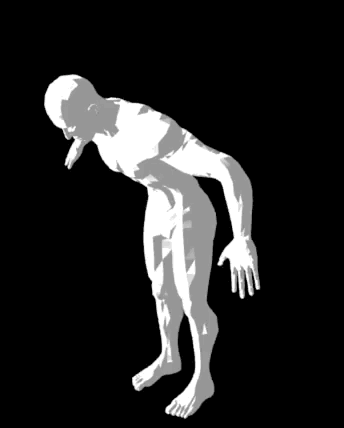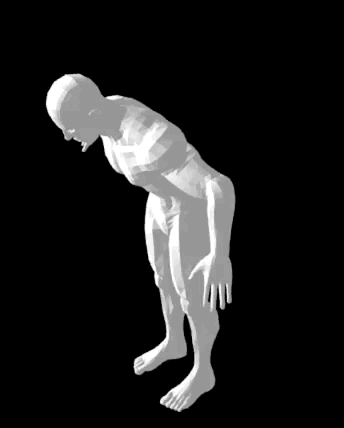One of the cornerstones of skeletal animation is a vertex shader that blends the influences of your model’s bones and applies this blended influence to your model’s vertices in order to move and animate them.

A “bone” is just a rotation and translation in model space, so there are many different ways to represent a bone.

The most common representation is as a transformation matrix that encodes a desired rotation and translation. In recent years, however, the Dual Quaternion has proven itself as a very useful alternative.

When I first started using dual quaternions I struggled to get the math working in my vertex shaders. Most of the papers that I found explained the concepts, but didn’t provide a reference vertex shader.

So the goal of this post is to be a reference that I wish that I had while I was learning. It’s meant for the next person in my shoes that gets stuck while implementing dual quaternion linear blending.

In this post we’ll step through a dual quaternion vertex shader and explain how it works.

## Why use Dual Quaternions?

There are a few benefits to using dual quaternions, but the one that first pulled my interest was how well suited they are for shortest path rotation interpolation.Interpolating bones via matricesInterpolating bones via dual quaternions

Above are two side-by-side gifs of a human rig bending forward.

The left rig is represented using matrices. The right gif takes the left gif’s matrices and converts them into dual quaternions using mat4-to-dual-quat.

You can see that the left rigs torso stretches as it bends, but the right rig remains rigid.

This is because when you interpolate matrices you are not guaranteed to have a rigid transform or to interpolate rotations along the shortest path.

In contrast, interpolating rotations via dual quaternions will always guarantee a rigid transform and a shortest path rotation. Hence the perfectly rigid bending of the torso.

If you want to explore further, here’s how to view both demo’s locally:

``````git clone https://github.com/chinedufn\
npm install

# Run the matrix interpolation demo
git checkout fe7cba26
npm run demo

# Run the dual quaternion interpolation demo
git checkout 02cfd8
npm run demo
``````

With that quick background out of the way, let’s break down an example vertex shader for dual quaternion based skeletal animation (dual quaternion linear blending).

Below is our entire dual quaternion linear blending vertex shader. Note that it isn’t very optimized and we’re creating more variables within the shader than we really need. That’s fine, we just want to understand the core concepts.

``````attribute vec3 aVertexPosition;
attribute vec3 aVertexNormal;
attribute vec4 aJointIndex;
attribute vec4 aJointWeight;

uniform mat4 uMVMatrix;
uniform mat4 uPMatrix;
uniform mat3 uNMatrix;
uniform vec4 boneRotQuaternions;
uniform vec4 boneTransQuaternions;

void main (void) {
vec4 weightedRotQuats =
boneRotQuaternions[int(aJointIndex.x)] * aJointWeight.x +
boneRotQuaternions[int(aJointIndex.y)] * aJointWeight.y +
boneRotQuaternions[int(aJointIndex.z)] * aJointWeight.z +
boneRotQuaternions[int(aJointIndex.w)] * aJointWeight.w;

vec4 weightedTransQuats =
boneTransQuaternions[int(aJointIndex.x)] * aJointWeight.x +
boneTransQuaternions[int(aJointIndex.y)] * aJointWeight.y +
boneTransQuaternions[int(aJointIndex.z)] * aJointWeight.z +
boneTransQuaternions[int(aJointIndex.w)] * aJointWeight.w;

float xRot = weightedRotQuat;
float yRot = weightedRotQuat;
float zRot = weightedRotQuat;
float wRot = weightedRotQuat;

float rotQuatMagnitude = sqrt(xRot * xRot + yRot * yRot
+ zRot * zRot + wRot * wRot);
weightedRotQuat = weightedRotQuat / rotQuatMagnitude;
weightedTransQuat = weightedTransQuat / rotQuatMagnitude;

float xR = weightedRotQuat;
float yR = weightedRotQuat;
float zR = weightedRotQuat;
float wR = weightedRotQuat;
float xT = weightedTransQuat;
float yT = weightedTransQuat;
float zT = weightedTransQuat;
float wT = weightedTransQuat;
float t0 = 2.0 * (-wT * xR + xT * wR - yT * zR + zT * yR);
float t1 = 2.0 * (-wT * yR + xT * zR + yT * wR - zT * xR);
float t2 = 2.0 * (-wT * zR - xT * yR + yT * xR + zT * wR);
mat4 weightedJointMatrix = mat4(
1.0 - (2.0 * yR * yR) - (2.0 * zR * zR),
(2.0 * xR * yR) + (2.0 * wR * zR),
(2.0 * xR * zR) - (2.0 * wR * yR),
0,
(2.0 * xR * yR) - (2.0 * wR * zR),
1.0 - (2.0 * xR * xR) - (2.0 * zR * zR),
(2.0 * yR * zR) + (2.0 * wR * xR),
0,
(2.0 * xR * zR) + (2.0 * wR * yR),
(2.0 * yR * zR) - (2.0 * wR * xR),
1.0 - (2.0 * xR * xR) - (2.0 * yR * yR),
0,
t0,
t1,
t2,
1
);

gl_Position = uPMatrix * uMVMatrix *
weightedJointMatrix * vec4(aVertexPosition, 1.0);

vec3 blendedNormal = uNMatrix *
(weightedMatrix * vec4(aVertexNormal, 0.0)).xyz;
}
``````

Take one more moment to scan through the entire shader again, then we’ll break it down piece by piece.

## Breaking it down

We begin by defining our attributes. `aVertexPosition` and `aVertexNormal` hold the positions and normals for the mesh that we’re animating.

``````attribute vec3 aVertexPosition;
attribute vec3 aVertexNormal;
attribute vec4 aJointIndex;
attribute vec4 aJointWeight;
``````

When skinning, you’ll usually allow each vertex to be influenced by up to three or four different bones. In our shader we’re allowing a maximum of four bones to influence a vertex, hence `aJointIndex` and `aJointWeight` being `vec4`s.

`aJointIndex` holds up to four numbers between `0` - `17` that correspond to one of our 18 bones.

`aJointWeight` encodes the weighting of each bone, or the amount that it should affect our vertex.

For example - let’s look at a set of joint indices and weights.

``````// Example values for a vertex
aJointIndex = [0, 13, 5, 1]
aJointWeight = [0.6, 0.2, 0.1, 0.1]
``````

In this example our vertex is being affected 60% by bone zero, 20% by bone thirteen, ten% by bone 5 and 10% by bone 1. So if bone zero is moved of rotated, 60% of that transformation ends up being applied to our vertex.

Next we define our uniforms.

``````uniform mat4 uMVMatrix;
uniform mat4 uPMatrix;
uniform mat3 uNMatrix;
uniform vec4 boneRotQuaternions;
uniform vec4 boneTransQuaternions;
``````

`uMVMatrix` and `uPMatrix` are our model view matrix and perspective matrix. These are used to know where to draw our model in our 3d world. `uNMatrix` is used to transform our normals as our model rotates.

We encode the rotational and translation components of our dual quaternions into `boneRotQuaternions` and `boneTransQuaternions`. We split our dual quaternion into two components because OpenGL ES 2.0 doesn’t support 2x4 matrices (which would have held both the four rotational and four translational values in a dual quaternion).

Since our model has 18 bones, we make our dual quaternion arrays hold 18 sets of 8 values.

Next we blend the dual quaternions that affect this vertex.

``````void main (void) {
vec4 weightedRotQuats =
boneRotQuaternions[int(aJointIndex.x)] * aJointWeight.x +
boneRotQuaternions[int(aJointIndex.y)] * aJointWeight.y +
boneRotQuaternions[int(aJointIndex.z)] * aJointWeight.z +
boneRotQuaternions[int(aJointIndex.w)] * aJointWeight.w;

vec4 weightedTransQuats =
boneTransQuaternions[int(aJointIndex.x)] * aJointWeight.x +
boneTransQuaternions[int(aJointIndex.y)] * aJointWeight.y +
boneTransQuaternions[int(aJointIndex.z)] * aJointWeight.z +
boneTransQuaternions[int(aJointIndex.w)] * aJointWeight.w;
``````

Lines like `boneRotQuaternions[int(aJointIndex.x)] * aJointWeight.x` are saying “get the rotation component of the dual quaternion of the joint that corresponds do this index and then multiply it by the weighting for this joint.

Once we add all of these weightings up we have our final blended dual quaternion.

One beautiful thing about dual quaternions is that blending (combining) them really is this simple. We just add up the influences of each joint and we’re done.

Now before we move forward we normalize our dual quaternion. This ensures that we have a rigid transform, which might not have been the case after blending our dual quaternions.

Basically, if you don’t do this you will see very unpleasant artifacts.

``````  float xRot = weightedRotQuat;
float yRot = weightedRotQuat;
float zRot = weightedRotQuat;
float wRot = weightedRotQuat;

float rotQuatMagnitude = sqrt(xRot * xRot + yRot * yRot
+ zRot * zRot + wRot * wRot);
weightedRotQuat = weightedRotQuat / rotQuatMagnitude;
weightedTransQuat = weightedTransQuat / rotQuatMagnitude;
``````

I created a bunch of two letter floats here, but if you look passed that all we’re doing is turning our dual quaternion into a 4x4 matrix. This makes it very easy to transform our vertex using our newly blended joint matrix since `matrix * vertex` multiplication is already built into GLSL.

``````  float xR = weightedRotQuat;
float yR = weightedRotQuat;
float zR = weightedRotQuat;
float wR = weightedRotQuat;
float xT = weightedTransQuat;
float yT = weightedTransQuat;
float zT = weightedTransQuat;
float wT = weightedTransQuat;
float t0 = 2.0 * (-wT * xR + xT * wR - yT * zR + zT * yR);
float t1 = 2.0 * (-wT * yR + xT * zR + yT * wR - zT * xR);
float t2 = 2.0 * (-wT * zR - xT * yR + yT * xR + zT * wR);
mat4 weightedJointMatrix = mat4(
1.0 - (2.0 * yR * yR) - (2.0 * zR * zR),
(2.0 * xR * yR) + (2.0 * wR * zR),
(2.0 * xR * zR) - (2.0 * wR * yR),
0,
(2.0 * xR * yR) - (2.0 * wR * zR),
1.0 - (2.0 * xR * xR) - (2.0 * zR * zR),
(2.0 * yR * zR) + (2.0 * wR * xR),
0,
(2.0 * xR * zR) + (2.0 * wR * yR),
(2.0 * yR * zR) - (2.0 * wR * xR),
1.0 - (2.0 * xR * xR) - (2.0 * yR * yR),
0,
t0,
t1,
t2,
1
);
``````

As a quick side note - You could also just transform your vertex’s position using your dual quaternion, instead of converting it into a matrix first. While writing this tutorial I found an example dual quaternion shader without any matrix conversions. If I ever run into performance issues with converting to a matrix here I’ll give this dual quaternion only approach a try.

Lastly we get our `gl_Position` by multiplying our perspective, model view and blended joint matrix with our original vertex position.

``````  gl_Position = uPMatrix * uMVMatrix *
weightedJointMatrix * vec4(aVertexPosition, 1.0);

vec3 blendedNormal = uNMatrix *
(weightedMatrix * vec4(aVertexNormal, 0.0)).xyz;
}
``````